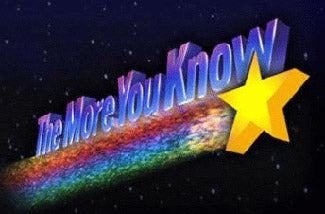Email: Sales@IPGparts.com | Phone: 407-324-4684
Email: Sales@IPGparts.com | Phone: 407-324-4684

# Spring Conversion Chart -- kg/mm to lbs/in

When I find useful information on the internet I like to try and pass it on. Ever gotten irritated trying to figure out what your kg/mm spring rates are in lbs/in ? I came across this handy post on Evolutionm.net --

1lb = 0.45359237kg

1in = 25.4mm .45359237 / 25.4 = .017857967322834645669291338582677

So now we know that 1lb/in = .017857967322834645669291338582677.

So if we want a spring that's roughly 560lbs; we'd multiply both sides by 560, and come up with roughly a 10k spring rate. Alternatively if you already know the kg/mm rate and want to find out what the lbs/in is, just divide the spring rate by that reaaally long factoral above (the .0178...).

But just in case you all didn't want to go through all that, here's a chart:

kg/mm to lbs/in -----------------------------

16 = 896

15 = 840

14 = 784

13 = 728

12 = 672

11 = 616

10 = 560

9.0 = 504

8.5 = 476

8.0 = 448

7.5 = 420

7.0 = 392

6.5 = 364

6.0 = 336

5.5 = 308

5.0 = 280

4.5 = 252

4.0 = 224

3.0 = 168

2.0 = 112